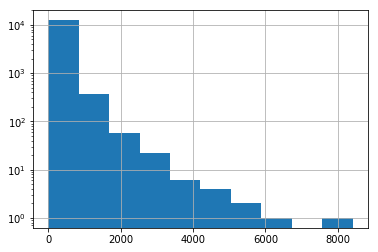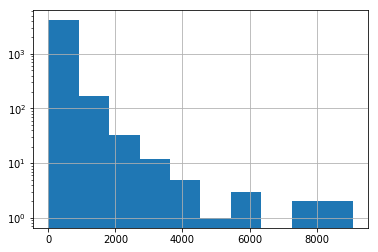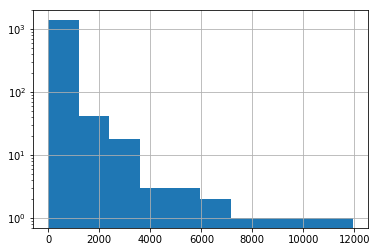import pandas as pd
import matplotlib.pyplot as plt
import datetime
import numpy as np
%matplotlib inline

from scipy.stats import shapiro
from scipy.stats import normaltest
from scipy.stats import anderson

# Input: series has the sample whose distribution we want to test
# Output: gaussian boolean True if it is normal distribution and False otherwise.
def testNormality(series):

alpha = 0.05
gaussian = False

# only if the three tests give normal will be normal. If we find one that is not passed, then it is NOT normal.

# Shapiro-Wilk Test - for smaller data sets around thousands of records
print('length of series in Shapiro is: '+ str(len(series)))
stats1, p1 = shapiro(series)
print('Statistics Shapiro-Wilk Test =%.3f, p=%.3f' % (stats1, p1))
if p1 > alpha:
gaussian = True
print('Shapiro.Wilk says it is Normal '+ str(gaussian))

gaussian = False # because of intermediate printing, reinitialize
# D'Agostino and Pearson's Test
stats2, p2 = normaltest(series) #dataw.humid
print('Statistics D\'Agostino and Pearson\'s Test=%.3f, p=%.3f' % (stats2, p2))
if p2 > alpha:
gaussian = False
print('D\'Agostino and Pearson\'s says it is Normal '+ str(gaussian))

# Anderson-Darling Test
'''result = anderson(series)
print('Statistic: %.3f' % result.statistic)
for i in range(len(result.critical_values)):
sl, cv = result.significance_level[i], result.critical_values[i]
if result.statistic > result.critical_values[i]:
gaussian = False'''

return gaussian


from scipy.stats import ttest_ind
from scipy.stats import mannwhitneyu

# Input:
# series1 is the series with the set of measurements for every single worker in case A of controlled experiment
# series2 is the series with the set of measurements for every single worker in case B of controlled experiment
# gaussian is the boolean value indicating if the samples have passed the test of normality or not (True is apply parametric test)
# Output:
# stats of statistical test
# p-value
# acceptHo (True if we fail to reject it and False if we reject it)
def compareTwoSamples(series1,series2, gaussian):
# Tests to compare two samples (H0: they have equal distribution; H1: they have different distribution)

alpha = 0.05
acceptH0 = False

if (gaussian == True):
# Run Student's T-test
stats, p = ttest_ind(series1, series2)
print('Statistics=%.3f, p=%.3f' % (stats, p))

else:

# Run Mann-Whitney; Kruskal-Wallis test is for more samples.
stats, p = mannwhitneyu(series1, series2)
print('Statistics=%.3f, p=%.3f' % (stats, p))

# result - hypothesis testing

if p > alpha:
acceptH0 = True

print('The two samples have the same distribution (meanA = meanB): ' + str(acceptH0))
return stats,p,acceptH0


#https://www.kaggle.com/uchayder/likes-shares-comments-and-mann-whitney-u-test

posts = pd.read_csv('post.csv')

com_count = comments.groupby('pid').count()['cid']
data = posts.join(com_count,on='pid', rsuffix='c')[['msg', 'likes', 'shares', 'cid', 'gid']]
data.columns = ['msg', 'likes', 'shares', 'comments', 'gid']
data['msg_len'] = data.apply(lambda x: len(str(x['msg'])),axis=1)

msg likes shares comments gid msg_len
0 O.K whats the deal with this Poison Ivy Rash t... NaN 1 51.0 117291968282998 615
1 A friend of mine just told me that the sewer s... NaN 0 6.0 117291968282998 116
2 We are looking forward someone to tear down an... NaN 0 9.0 117291968282998 183
3 Need a small section of stucco replaced on the... NaN 0 4.0 117291968282998 100
4 Can anybody recommend a good cheap plumber or ... NaN 0 9.0 117291968282998 56
data.gid = data.gid.map({117291968282998: 1, 25160801076: 2, 1443890352589739: 3})

data.fillna(0,inplace=True)

msg likes shares comments gid msg_len
0 O.K whats the deal with this Poison Ivy Rash t... 0.0 1 51.0 1.0 615
1 A friend of mine just told me that the sewer s... 0.0 0 6.0 1.0 116
2 We are looking forward someone to tear down an... 0.0 0 9.0 1.0 183
3 Need a small section of stucco replaced on the... 0.0 0 4.0 1.0 100
4 Can anybody recommend a good cheap plumber or ... 0.0 0 9.0 1.0 56
group1 = data[data['gid']==1.0]
group2 = data[data['gid']==2.0]
group3 = data[data['gid']==3.0]

norm_g1 = testNormality(group1['msg_len'])
norm_g2 = testNormality(group2['msg_len'])
norm_g3 = testNormality(group3['msg_len'])

length of series in Shapiro is: 12897
Statistics Shapiro-Wilk Test =0.535, p=0.000
Shapiro.Wilk says it is Normal False
Statistics D'Agostino and Pearson's Test=16534.036, p=0.000
length of series in Shapiro is: 4327
Statistics Shapiro-Wilk Test =0.467, p=0.000
Shapiro.Wilk says it is Normal False
Statistics D'Agostino and Pearson's Test=5887.198, p=0.000
length of series in Shapiro is: 1456
Statistics Shapiro-Wilk Test =0.371, p=0.000
Shapiro.Wilk says it is Normal False
Statistics D'Agostino and Pearson's Test=2000.684, p=0.000

/srv/paws/lib/python3.6/site-packages/scipy/stats/morestats.py:1309: UserWarning: p-value may not be accurate for N > 5000.
warnings.warn("p-value may not be accurate for N > 5000.")

group1['msg_len'].hist(log=True)

<matplotlib.axes._subplots.AxesSubplot at 0x7f574566d0f0>group2['msg_len'].hist(log=True)

<matplotlib.axes._subplots.AxesSubplot at 0x7f5744812c18>group3['msg_len'].hist(log=True)

<matplotlib.axes._subplots.AxesSubplot at 0x7f5747c027f0>normal = norm_g1 and norm_g2
stats,p,acceptH0 = compareTwoSamples(group1['msg_len'], group2['msg_len'], normal )
corrected_p = multipletests(p,
alpha = 0.05,
method = 'holm')
print(corrected_p)
print('p > 0.05 is accept so with this correction is False as in the blog')

Statistics=26059615.000, p=0.000
The two samples have the same distribution (meanA = meanB): False
(array([ True]), array([3.70914016e-11]), 0.050000000000000044, 0.05)
p > 0.05 is accept so with this correction is False as in the blog

# tested my code and these samples give the same results as in the blog post - see Conclusions 4 for G1-G2 and G1-G3 but not G2-G3 (but there was also an error in the code!)
# code of the correction package https://www.statsmodels.org/dev/_modules/statsmodels/stats/multitest.html

#!pip install statsmodels
from statsmodels.sandbox.stats.multicomp import multipletests
# it returns
'''    reject : array, boolean
true for hypothesis that can be rejected for given alpha
pvals_corrected : array
p-values corrected for multiple tests
alphacSidak: float
corrected alpha for Sidak method
alphacBonf: float
corrected alpha for Bonferroni method '''

normal = norm_g2 and norm_g3
stats,p,acceptH0 = compareTwoSamples(group2['msg_len'], group3['msg_len'], normal )
corrected_p = multipletests(p,
alpha = 0.05,
method = 'holm')
print(corrected_p)
print('p > 0.05 is accept so with this correction is False as in the blog')

Statistics=3073382.500, p=0.082
The two samples have the same distribution (meanA = meanB): True
(array([False]), array([0.08205476]), 0.050000000000000044, 0.05)

normal = norm_g1 and norm_g3
stats,p,acceptH0 = compareTwoSamples(group1['msg_len'], group3['msg_len'], normal)
corrected_p = multipletests(p,
alpha = 0.05,
method = 'holm')
print(corrected_p)
print('p > 0.05 is accept so with this correction is False as in the blog')

Statistics=9030824.500, p=0.008
The two samples have the same distribution (meanA = meanB): False

(9030824.5, 0.008424448276112752, False)
rejected, p_corrected, a1, a2 = multipletests(comparison.p_value,
alpha = 0.05,
method = 'holm')

# https://gist.github.com/naturale0/3915e2def589553e91dce99e69d138cc